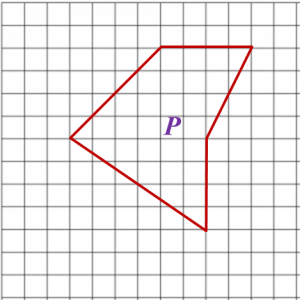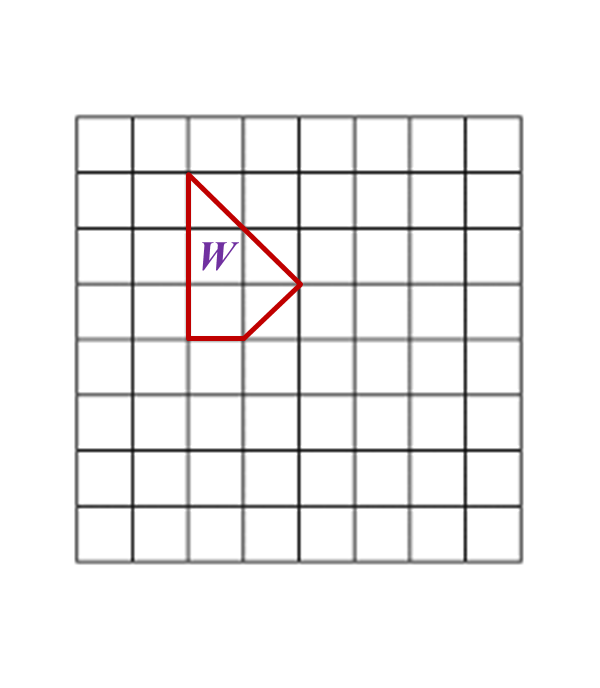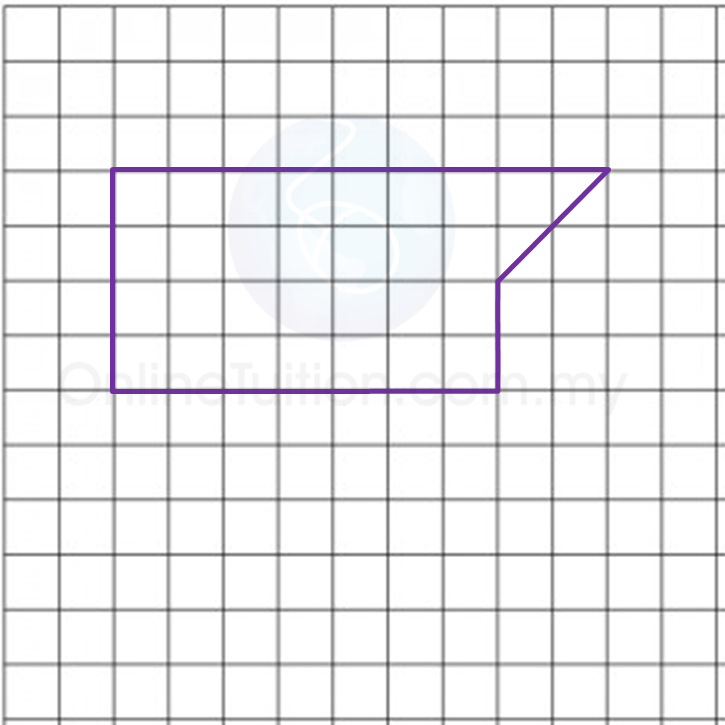# 4.2.1 Scale Drawings, PT3 Focus Practice

Question 1:
Diagram below shows polygon P drawn on a grid of equal squares with sides of 1 unit.On the grid in the answer space, redraw polygon P using the scale
The grid has equal squares with sides of 1 unit.Question 2:
Diagram below shows polygon W drawn on a grid of equal squares with sides of 1 unit.On the grid in the answer space, redraw polygon W using the scale 1 : 2.
The grid has equal squares with sides of 1 unit.Question 3:
Diagram below shows a polygon.On the grid in the answer space, redraw the polygon using the scale 1 : 500. The grid has equal squares with sides of 1 cm.